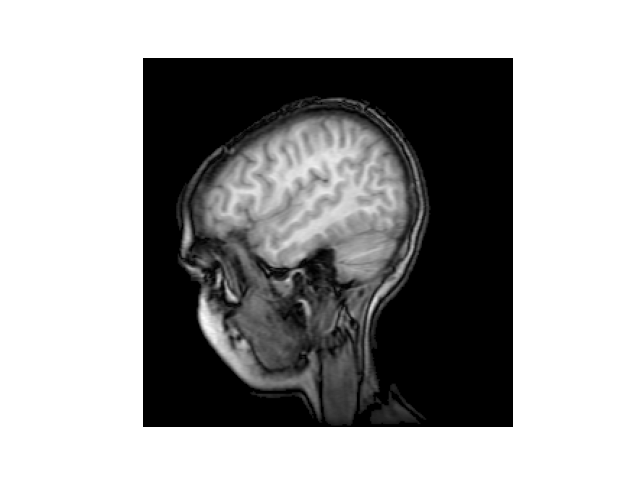MRI¶

This example illustrates how to read an image (of an MRI) into a NumPy array, and display it in greyscale using imshow.import matplotlib.pyplot as plt
import matplotlib.cbook as cbook
import numpy as np

# Data are 256x256 16 bit integers.
with cbook.get_sample_data('s1045.ima.gz') as dfile: